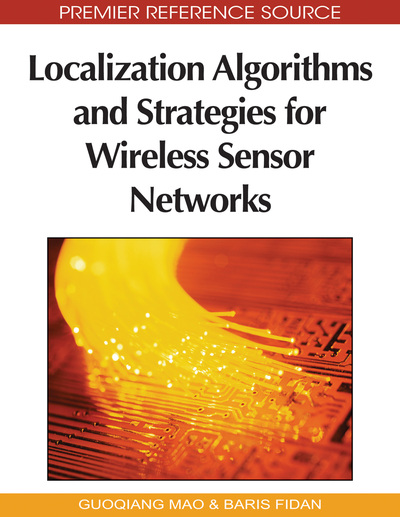# Sequential Localization with Inaccurate Measurements

Jia Fang (Yale University, USA), Dominique Duncan (Yale University, USA) and A. Stephen Morse (Yale University, USA)
DOI: 10.4018/978-1-60566-396-8.ch007

## Abstract

The sensor network localization problem with distance information is to determine the positions of all sensors in a network given the positions of some sensors and the distances between some pairs of sensors. In this chapter the authors present a sequential algorithm for estimating sensor positions when only inaccurate distance measurements are available, and they use experimental evaluation to demonstrate network instances on which the algorithm is effective.
Chapter Preview
Top

## Introduction

In many situations where wireless sensor networks are used, only the positions of some of the sensors are known, and the positions of the remaining sensors must be inferred from the known locations and available inter-sensor distance measurements. More formally, consider n sensors in the plane labelled 1 through n, where the positions of some sensors are known, and the measured distances between some pairs of sensors are known. The sensors with known positions are called anchors. Since ranging devices are never exact, we consider the following model for the type of distance measurements obtained. For each inter-sensor distance measurement, we assume that an accuracy guarantee denoted by ε > 0 ofis given such that the actual inter-sensor distance is within ε of the measured distance. Obviously, sensor positions are generally not uniquely determined by inaccurate distance measurements. In Moore et al.(2004) and Priyantha et al. (2003), it is pointed out that it is more important to obtain position estimates which reflect the general layout of the actual sensor positions, rather than simply position estimates which induce inter-sensor distances within some desired tolerance of the given inter-sensor distance measurements. In Priyantha et al. (2003), a modified spring based relaxation method is used to obtain sensor estimates, and it is shown via experimental evaluations that the estimated positions reflect the general layout of the actual sensor positions. Our work is most closely related to Moore et al. (2004) where the aim was to compute position estimates for subnetworks called “robust quadrilaterals” with correctness guarantees. More specifically, an algorithm is given which assigns position estimates to the sensors in a robust quadrilateral only if the position estimates can be guaranteed to be free of “flip ambiguities” with high probability Moore et al. (2004).

Roughly speaking, position estimates are desired so that estimated positions are approximately congruent to the actual positions. We capture this notion using the concept of “correctly oriented” position estimates which we define as follows. For two points p and q in R2, let l(p,q) denote the line segment with endpoints p and q. For m≥ 4 sensors labelled 1 through m and, let pi denote the position of sensor i, and let qi denote the estimated position of sensor i. The estimated positions q1,..., qm are said to be correctly oriented if for all distinct, the line segments l(pi,, pj) and l(pk, pl) intersect if and only if the line segments l(qi,, qj) and l(qk, ql) intersect. As an illustration, suppose sensors 1, 2, 3 and 4 are positioned at p1, p2, p3 and p4 respectively as shown in Figure 1(a) and 1(b). For each sensor i, let qi and q’i denote two estimated positions of sensor i. Suppose qi for i=1,2,3,4 are as shown in Figure 1(a), and q’i are as shown in Figure 1(b). It is easy to see that {q1, q2, q3, q4} are correctly oriented while {q’1, q’2, q’3, q’4} are not correctly oriented.

## Complete Chapter List

Search this Book:
Reset